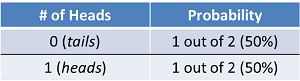# Definition of Probability

## Definition of probability

You have to complete Definition of various terms Exam First to attempt this Exam

## Probability

Probability is the likelihood that an event will occur and is calculated by dividing the number of favorable outcomes by the total number of possible outcomes. The simplest example is a coin flip. When you flip a coin there are only two possible outcomes, the result is either heads or tails. And so, the probability of getting heads is 1 out of 2, or ½, or 50%. The table below shows the distribution of the probability of each outcome. There is a 50% chance the outcome will be heads, and there is a 50% chance the outcome will be tails.## Probability Distribution

Probability distribution maps out the likelihood of multiple outcomes in a table or equation. If we go back to the coin flip example, we already know that one flip of the coin has only two possible outcomes. But if we flip the coin twice in a row, there are four possible outcomes (heads-heads, heads-tails, tails-heads and tails-tails). So now that we have a series of potential outcomes, consider the probabilities of getting heads once, twice or zero times.